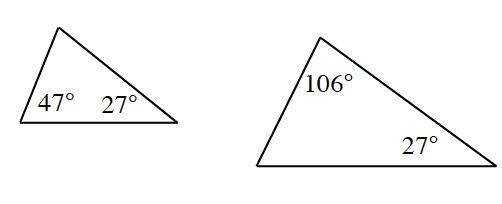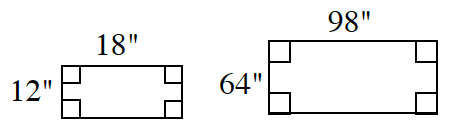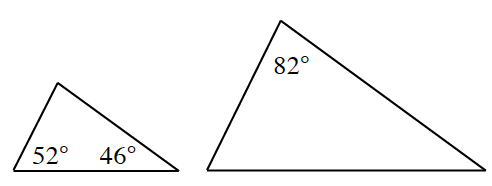### Home > GC > Chapter 4 > Lesson 4.1.2 > Problem4-18

4-18.

Examine each pair of figures below. Are they similar? Explain how you know.

1.Use the Triangle Angle Sum Theorem to determine whether or not at least two of the angles from each triangle are congruent.

1.Check the ratios of the corresponding sides to see if they are equal.

1.There is not enough information about the angles in the large triangle to determine whether or not the triangles are similar.